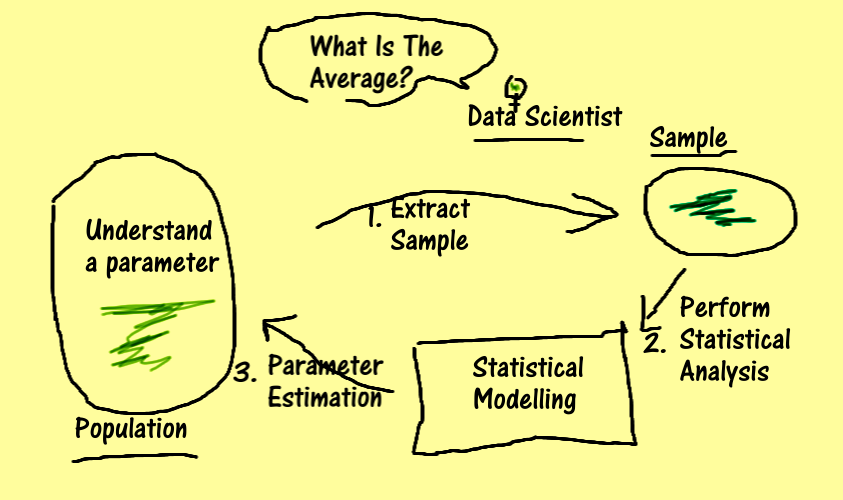### Statistical Inference - SI401 - Sem.2 - 20/21This course will provide undergraduate students with broad based knowledge in statistical inference. Topics include confidence intervals, tests of significance, chi-square tests of goodness-of-fit and independence, regression analysis, and analysis of variance. Students will be introduced to a standard computer statistical package. Fundamental concepts of statistics. Point estimation, maximum likelihood estimators, hypothesis testing, confidence regions, t-test, analysis of variance, non-parametric tests, chi-square goodness-of-fit tests, correlation, regression analysis, and selected additional topics.